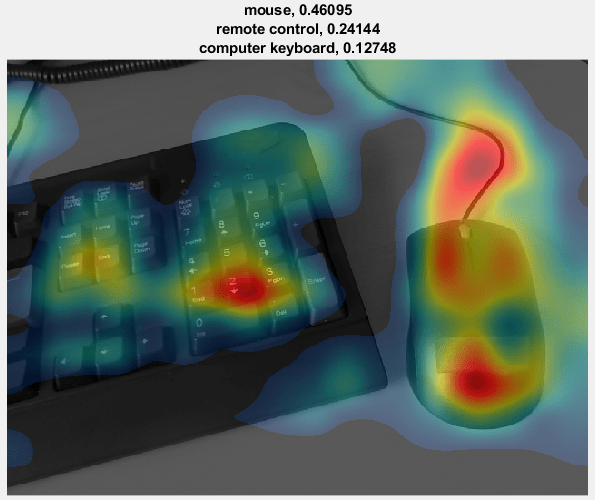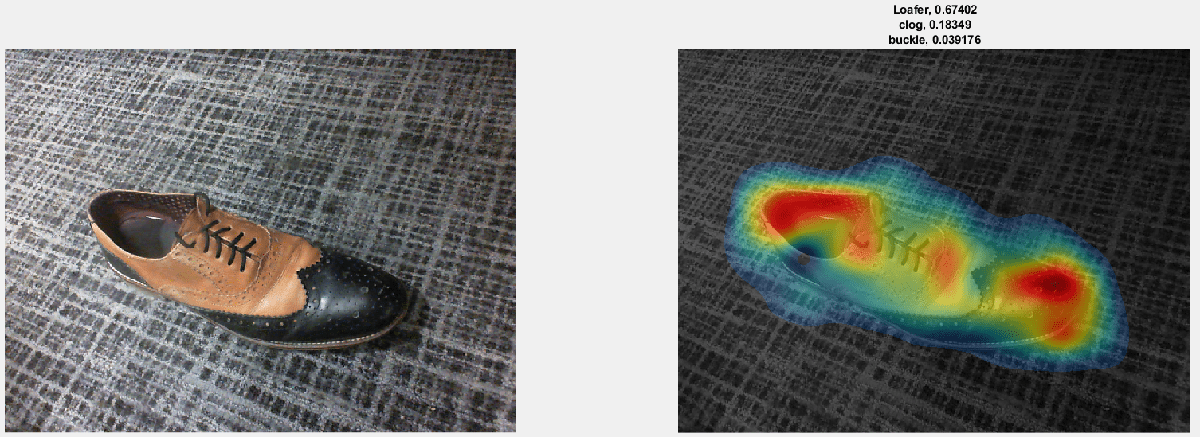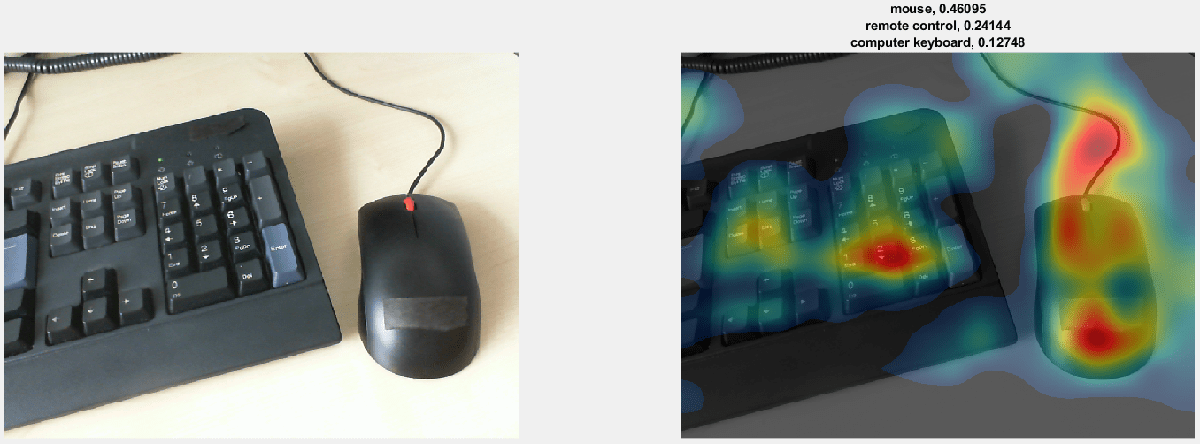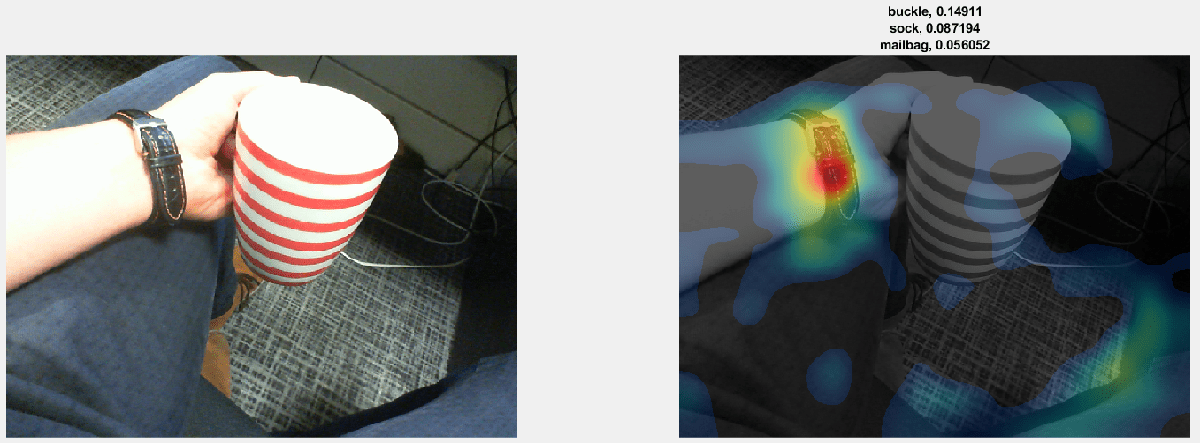Documentation

## Investigate Network Predictions Using Class Activation Mapping

This example shows how to use class activation mapping (CAM) to investigate and explain the predictions of a deep convolutional neural network for image classification.

Deep learning networks are often considered to be "black boxes" that offer no way of figuring out what a network has learned or which part of an input to the network was responsible for the prediction of the network. When these models fail and give incorrect predictions, they often fail spectacularly without any warning or explanation. Class activation mapping  is one technique that you can use to get visual explanations of the predictions of convolutional neural networks. Incorrect, seemingly unreasonable predictions can often have reasonable explanations. Using class activation mapping, you can check if a specific part of an input image "confused" the network and led it to make an incorrect prediction.

You can use class activation mapping to identify bias in the training set and increase model accuracy. If you discover that the network bases predictions on the wrong features, then you can make the network more robust by collecting better data. For example, suppose that you train a network to distinguish images of cats and dogs. The network has high accuracy on the training set, but performs poorly on real-world examples. By using class activation mapping on the training examples, you discover that the network is basing predictions not on the cats and dogs in the images, but on the backgrounds. You then realize that all your cat pictures have red backgrounds, all your dog pictures have green backgrounds, and that it is the color of the background that the network learned during training. You can then collect new data that does not have this bias.

This example class activation map shows which regions of the input image contribute the most to the predicted class `mouse`. Red regions contribute the most.### Load Pretrained Network and Webcam

Load a pretrained convolutional neural network for image classification. SqueezeNet, GoogLeNet, ResNet-18, and MobileNet-v2 are relatively fast networks. SqueezeNet is the fastest network and its class activation map has four times higher resolution than the maps of the other networks. You cannot use class activation mapping with networks that have multiple fully connected layers at the end of the network, such as AlexNet, VGG-16, and VGG-19.

```netName = "squeezenet"; net = eval(netName);```

Create a `webcam` object and connect to your webcam.

`camera = webcam;`

Extract the image input size and the output classes of the network. The `activationLayerName` helper function, defined at the end of this example, returns the name of the layer to extract the activations from. This layer is the ReLU layer that follows the last convolutional layer of the network.

```inputSize = net.Layers(1).InputSize(1:2); classes = net.Layers(end).Classes; layerName = activationLayerName(netName);```

### Display Class Activation Maps

Create a figure and perform class activation mapping in a loop. To terminate execution of the loop, close the figure.

```h = figure('Units','normalized','Position',[0.05 0.05 0.9 0.8],'Visible','on'); while ishandle(h)```

Take a snapshot using the webcam. Resize the image so that the length of its shortest side (in this case, the image height) equals the image input size of the network. As you resize, preserve the aspect ratio of the image. You can also resize the image to a larger or smaller size. Making the image larger increases the resolution of the final class activation map, but can lead to less accurate overall predictions.

Compute the activations of the resized image in the ReLU layer that follows the last convolutional layer of the network.

``` im = snapshot(camera); imResized = imresize(im,[inputSize(1), NaN]); imageActivations = activations(net,imResized,layerName);```

The class activation map for a specific class is the activation map of the ReLU layer that follows the final convolutional layer, weighted by how much each activation contributes to the final score of that class. Those weights equal the weights of the final fully connected layer of the network for that class. SqueezeNet does not have a final fully connected layer. Instead, the output of the ReLU layer that follows the last convolutional layer is already the class activation map.

You can genereate a class activation map for any output class. For example, if the network makes an incorrect classification, you can compare the class activation maps for the true and predicted classes. For this example, generate the class activation map for the predicted class with the highest score.

``` scores = squeeze(mean(imageActivations,[1 2])); if netName ~= "squeezenet" fcWeights = net.Layers(end-2).Weights; fcBias = net.Layers(end-2).Bias; scores = fcWeights*scores + fcBias; [~,classIds] = maxk(scores,3); weightVector = shiftdim(fcWeights(classIds(1),:),-1); classActivationMap = sum(imageActivations.*weightVector,3); else [~,classIds] = maxk(scores,3); classActivationMap = imageActivations(:,:,classIds(1)); end ```

Calculate the top class labels and the final normalized class scores.

``` scores = exp(scores)/sum(exp(scores)); maxScores = scores(classIds); labels = classes(classIds);```

Plot the class activation map. Display the original image in the first subplot. In the second subplot, use the `CAMshow` helper function, defined at the end of this example, to display the class activation map on top of a darkened grayscale version of the original image. Display the top three predicted labels with their predicted scores.

``` subplot(1,2,1) imshow(im) subplot(1,2,2) CAMshow(im,classActivationMap) title(string(labels) + ", " + string(maxScores)); drawnow end```

Clear the webcam object.

`clear camera`

### Example Maps

The network correctly identifies the object in this image as a loafer (a type of shoe). The class activation map in the image to the right shows the contribution of each region of the input image to the predicted class `Loafer`. Red regions contribute the most. The network bases its classifaction on the entire shoe, but the strongest input comes from the red areas – that is, the tip and the opening of the shoe.The network classifies this image as a mouse. As the class activation map shows, the prediction is based not only on the mouse in the image, but also the keyboard. Because the training set likely has many images of mice next to keyboards, the network predicts that images containing keyboards are more likely to contain mice.The network classifies this image of a coffee cup as a buckle. As the class activation map shows, the network misclassifies the image because the image contains too many confounding objects. The network detects and focuses on the watch wristband, not the coffee cup.### Helper Functions

`CAMshow(im,CAM)` overlays the class activation map `CAM` on a darkened, grayscale version of the image `im`. The function resizes the class activation map to the size of `im`, normalizes it, thresholds it from below, and visualizes it using a `jet` colormap.

```function CAMshow(im,CAM) imSize = size(im); CAM = imresize(CAM,imSize(1:2)); CAM = normalizeImage(CAM); CAM(CAM<0.2) = 0; cmap = jet(255).*linspace(0,1,255)'; CAM = ind2rgb(uint8(CAM*255),cmap)*255; combinedImage = double(rgb2gray(im))/2 + CAM; combinedImage = normalizeImage(combinedImage)*255; imshow(uint8(combinedImage)); end function N = normalizeImage(I) minimum = min(I(:)); maximum = max(I(:)); N = (I-minimum)/(maximum-minimum); end function layerName = activationLayerName(netName) if netName == "squeezenet" layerName = 'relu_conv10'; elseif netName == "googlenet" layerName = 'inception_5b-output'; elseif netName == "resnet18" layerName = 'res5b_relu'; elseif netName == "mobilenetv2" layerName = 'out_relu'; end end```

 Zhou, Bolei, Aditya Khosla, Agata Lapedriza, Aude Oliva, and Antonio Torralba. "Learning deep features for discriminative localization." In Proceedings of the IEEE Conference on Computer Vision and Pattern Recognition, pp. 2921-2929. 2016.

Download ebook